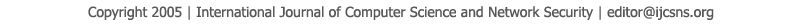To search, Click below search items.All Published Papers Search Service
 Title Author Year Keyword
 Title A Computer Oriented Solution for the Fractional Boundary Value Problem with Fuzzy Parameters with Application to Singular Perturbed Problems Author Somia A. Asklany and I.K.Youssef, Citation Vol. 21  No. 12  pp. 223-227 Abstract The papers use the finite difference technique to replace the continuous fractional order boundary value problem (BVP) with fuzzy boundary parameters into an equivalent fuzzy algebraic system. A decomposition of the inverted coefficient matrix into two parts (nonnegative and negative parts) and a corresponding reformulation of eight components of the fuzzy free terms are introduced. The treatment overcomes implementation difficulties associated with the extension principle also avoid the presence of singular matrices as well as the weak solutions appear in the embedding approach. Moreover, the treatment recovers the actual solution for some problems. Three simple standard numerical examples are considered to illustrate treatment. Also, different fractional orders of the BVP are considered (β =1.8, β=1.9, β=1.95) as well as the classical second order (β = 2). The calculated fuzzy solutions are compared with the fuzzy solutions of the classical singular perturbed BVP using triangular membership function. Keywords fractional order linear BVP, finite difference method, Fuzzy , Fuzzy linear systems. URL http://paper.ijcsns.org/07_book/202112/20211233.pdf